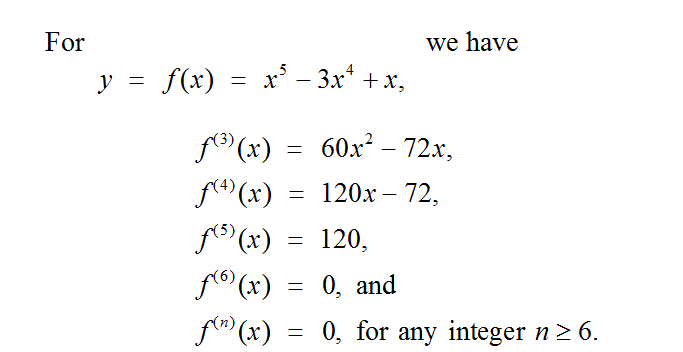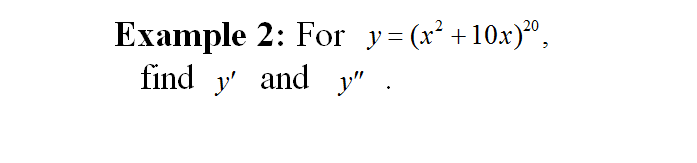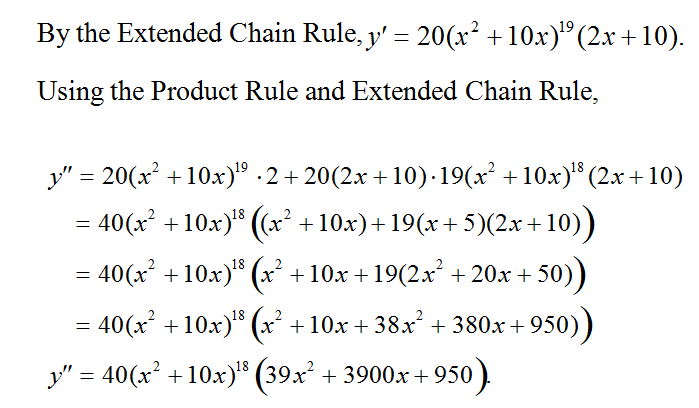# SCC Education

## Higher Order Derivatives & Concavity

Higher Order Derivatives  & Concavity,

Find derivatives of higher order,
Determine Concavity of a function,
Find the relative extreme of a function using the Second-Derivative Test,some-important-formulas-of-integration
solving-models-related-to-ordinary differential equation
differentiation-of-exponential-functions
area-between-curve
vector
application-of-derivatives
homogenous-differential-equation
inverse-trigonometry-questions
integration
integration-questions
Rolles-theorem-and-mean-value-theorem
Techniques-of-integration-substitution
critical-points-and-point-of-inflection

intermediate-value-theorem